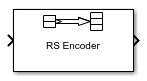Main Content

# Integer-Input RS Encoder

Create Reed-Solomon code from integer vector data

• Library:
• Communications Toolbox / Error Detection and Correction / Block

•## Description

The Integer-Input RS Encoder block creates a Reed-Solomon code.

The symbols for the code are integers between 0 and 2M-1, which represent elements of the finite field GF(2M). The default value of M is the smallest integer that is greater than or equal to log2(N+1), that is, `ceil(log2(N+1))`. You can change the default value of M by specifying the primitive polynomial for GF(2M), as described in Specify the Primitive Polynomial below. Restrictions on M and N are described in Restrictions on M and the Codeword Length N.

The input and output are integer-valued signals that represent messages and codewords, respectively. For more information, see Input and Output Signal Length in RS Blocks.

An (N, K) Reed-Solomon code can correct up to `floor((N-K)/2)` symbol errors (not bit errors) in each codeword.

Suppose M = 3, N = 23-1 = 7, and K = 5. Then a message is a vector of length 5 whose entries are integers between 0 and 7. A corresponding codeword is a vector of length 7 whose entries are integers between 0 and 7. The following figure illustrates possible input and output signals to this block when Codeword length N is set to `7`, Message length K is set to `5`, and the default primitive and generator polynomials are used.## Ports

### Input

expand all

Message, specified as one of the following:

• When there is no message shortening, a (NC×K)-by-1 integer column vector.

• When there is message shortening, a (NC×S)-by-1 integer column vector.

NC is the number of message words, K is the Message length K, and S is the Shortened message length S.

Note

The number of decoded message words equals the number of codewords.

For more information, see Input and Output Signal Length in RS Blocks.

Data Types: `single` | `double` | `int8` | `int16` | `int32` | `uint8` | `uint16` | `uint32`

### Output

expand all

Reed-Solomon codeword, returned as an (NC×(NK + SP)-by-1 integer column vector. NC is the number of codewords, N is the Codeword length N, K is the Message length K, S is the Shortened message length S, P is the number of punctures per codeword.

For more information, see Input and Output Signal Length in RS Blocks.

Data Types: `single` | `double` | `int8` | `int16` | `int32` | `uint8` | `uint16` | `uint32`

For more information, see Supported Data Types.

## Parameters

expand all

Codeword length, specified as an integer.

For more information, see Restrictions on M and the Codeword Length N and Input and Output Signal Length in RS Blocks.

Message word length, specified as an integer in the range [1, N–2], where N is the codeword length.

Shortened message word length, specified as an integer, such that SK. When Shortened message length S < Message length K, the Reed-Solomon code is shortened.

You still specify N and K values for the full-length (N, K) code but the decoding is shortened to an (NK+S, S) code.

#### Dependencies

To enable this parameter, select .

Generator polynomial with values in the range [0 to 2M–1], in order of descending power, specified as one of the following:

• A polynomial character vector. For more information, see Character Representation of Polynomials.

• An integer row vector that represents the coefficients of the generator polynomial in order of descending power.

• An integer Galois row vector that represents the coefficients of the generator polynomial in order of descending power.

Each coefficient is an element of the Galois field defined by the primitive polynomial. For more information, see Specify the Generator Polynomial.

Example: `[1 3 1 2 3]`, which is equivalent to `rsgenpoly(7,3)`

#### Dependencies

To enable this parameter, select .

Primitive polynomial in order of descending power. This polynomial is of order M and defines the finite Galois field GF(2M) corresponding to the integers that form message words and codewords. Specify the primitive polynomial as one of the following:

For more information, see Specify the Primitive Polynomial.

Example: `'X^3 + X + 1'`, which is the primitive polynomial used for a (7,3) code, `de2bi(primpoly(3,'nodisplay'),'left-msb')`

#### Dependencies

To enable this parameter, select .

Puncture vector, specified as an (NK)-by-1 binary column vector. Element indices with `1`s represent data symbol indices that pass through the block unaltered. Element indices with `0`s represent data symbol indices that get punctured, or removed, from the data stream. For more information, see Puncturing and Erasures.

Note

If the encoder is processing multiple codewords per frame, then the same puncture pattern holds for all codewords.

#### Dependencies

To enable this parameter, select .

## Block Characteristics

 Data Types `double` | `integer` | `single` Multidimensional Signals `no` Variable-Size Signals `no`

expand all

## Algorithms

This object implements the algorithm, inputs, and outputs described in Algorithms for BCH and RS Errors-only Decoding.

## See Also

### Functions

Introduced before R2006a

## Support

#### Bridging Wireless Communications Design and Testing with MATLAB

Download white paper